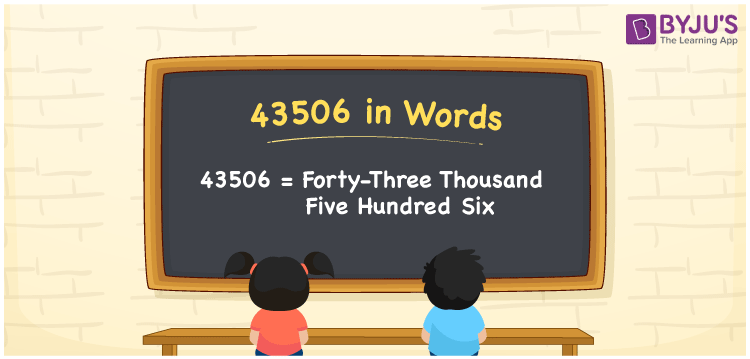# 43506 in words

43506 in words is written as Forty Three Thousand Five Hundred and Six. In 43506, 4 has a place value of ten thousand, 3 is in the place value of thousand, 5 is in the place value of hundred and 6 is in the place value of one. The article on Place Value gives more information. The number 43506 is used in expressions that relate to money, distance, length, social media views, and many more. For example, “There were Forty Three Thousand Five Hundred and Six casualties during the earthquake.”

 43506 in words Forty Three Thousand Five Hundred and Six Forty Three Thousand Five Hundred and Six in Numbers 43506

## 43506 in English Words## How to Write 43506 in Words?

We can convert 43506 to words using a place value chart. This can be done as follows. The number 43506 has 5 digits, so let’s make a chart that shows the place value up to 5 digits.

 Ten thousand Thousands Hundreds Tens Ones 4 3 5 0 6

Thus, we can write the expanded form as:

4 × Ten thousand + 3 × Thousand + 5 × Hundred + 0 × Ten + 6 × One

= 4 × 10000 + 3 × 1000 + 5 × 100 + 0 × 10 + 6 × 1

= 43506.

= Forty Three Thousand Five Hundred and Six.

43506 is the natural number that is succeeded by 43505 and preceded by 43507.

43506 in words – Forty Three Thousand Five Hundred and Six.

Is 43506 an odd number? – No.

Is 43506 an even number? – Yes.

Is 43506 a perfect square number? – No.

Is 43506 a perfect cube number? – No.

Is 43506 a prime number? – No.

Is 43506 a composite number? – Yes.

## Solved Example

1. Write the number 43506 in expanded form

Solution: 4 x 10000 + 3 x 1000 + 5 x 100 + 0 x 10 + 6 x 1

We can write 43506 = 40000 + 3000 + 500 + 0 + 6

= 4 x 10000 + 3 x 1000 + 5 x 100 + 0 x 10 + 6 x 1.

## Frequently Asked Questions on 43506 in words

Q1

### How to write the number 43506 in words?

43506 in words is written as Forty Three Thousand Five Hundred and Six.
Q2

### Is 43506 divisible by 3?

Yes. 43506 is divisible by 3.
Q3

### Is 43506 a prime number?

No. 43506 is not a prime number.